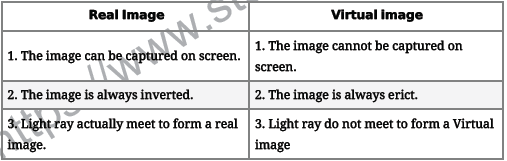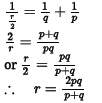# CBSE Class 10 Physics Light Reflection and Refraction Assignment Set B

Read and download free pdf of CBSE Class 10 Physics Light Assignment Set B. Get printable school Assignments for Class 10 Physics. Standard 10 students should practise questions and answers given here for Physics in Grade 10 which will help them to strengthen their understanding of all important topics. Students should also download free pdf of Printable Worksheets for Class 10 Physics prepared as per the latest books and syllabus issued by NCERT, CBSE, KVS and do problems daily to score better marks in tests and examinations

## Light Class 10 Physics Assignment Pdf

Class 10 Physics students should refer to the following printable assignment in Pdf for Light in standard 10. This test paper with questions and answers for Grade 10 Physics will be very useful for exams and help you to score good marks

### Class 10 Physics Assignment for Light

Question : In the set-up shown below, a clear image of a distant object is obtained on the screen. The focal length of the concave mirror is:a. 9.4 cm
b. 9.9 cm
c. 9.8 cm
d. 11.4 cm
Explanation: f = 11.8 - 2 = 9.8 cm

Question :  How does the frequency of a beam of ultra-violet light change when it goes from air into glass?
a. None of these
b. Frequency decreases
c. Remains the same
d. Frequency increases
Explanation: The frequency of light depends on the source of light and remains the same during refraction. The speed of the light changes when a ray of light passes from one medium to another. The speed of light is higher in a rarer medium than a denser medium. A ray of light travelling from a rarer medium to a denser medium slows down, due to which there is a change in the wave-length.

Question : What is the radius of curvature of the spherical mirror in the figure given, if PA = 10 cm?Answer :  We know that radius of curvature is twice the focal length f, so
R = 2f = 2(PA) = 2(10) = 20 cm

Question : Can a plane mirror be called a spherical mirror?
Answer :  Yes, a plane mirror can be called a spherical mirror because it also obeys the laws of reflection just like the spherical mirror . The only difference is that the reflecting surface of spherical mirror is curved while that of plane mirror is straight having infinite focal length and infinite centre of curvature. It can be called a spherical mirror of radius of curvature equal to infinity.

Question : Distinguish between real and virtual image in a lens.Question : If p, q and r denote the object distance, image distance and the radius of curvature respectively, of a spherical mirror, then find out the relation between them.
Answer :  From mirror formula, we have
1/f = 1/v + 1/u
Substitute the given values, we getwhich is the required relation between the three variables.

Question : An object 2 cm high is placed at a distance of 16 cm from a concave mirror which produces a real image 3 cm high.
1. Find the position of the image.
2. What is the focal length of mirror?
Object height, h= +2 cm
Image height, h' = - 3 cm (real image hence inverted)
Object distance, u = -16 cm
Image distance, v -?
Focal length, f=?
(i) Position of image
From the expression for magnification
m = h'/h = v/u
We have, v = -u h'/h
Putting values, we get v = -(-16 ) X -3/2
v = -24 cm
The image is formed at distance of 24 cm in front of the mirror (negative sign means object and image are on the same side).
(ii) Focal length of mirror
Using mirror formula, Putting values, we get
Using mirror formula,Q-1 An object is placed 30 cm from a convex lens. A real image is formed 20 cm from the lens. Find the focal length of the lens.

Q-2 A 2.0-cm-long pin is placed perpendicular to the principal axis of a convex lens of focal length 12 cm. The distance of the pin from the lens is 15 cm. Find the size of the image.

Q-3 Find the refractive index of glass with respect to water. The refractive indices of these with respect to air are 3/2 and 4/3 respectively.

Q-4 A point object is placed at a distance of 12 cm from a convex lens on its principal axis. Its image is formed on the other side of the lens at a distance of 18 cm from the lens.Find the focal length of the lens.

Q-5 The image of an object formed by a convex lens is of the same size as the object. If the image is formed at a distance of 40 cm, find the focal length of the lens. Also find the power of the lens. At what distance from the lens is the object placed?

Q-6 An object is placed on the principal axis of a concave lens at a distance of 20 cm from it. If the focal length of the lens is also 20 cm, find the location of the image.

Q-7 A beam of light traveling parallel to the principal axis of a concave lens appears to diverge from a point 20 cm behind the lens after passing through the lens. Find the power of the lens.

Q-8 A convex lens of power 4 D is placed at a distance of 40 cm from a wall. At what distance from the lens should a candle placed so that its image is formed on the wall?

Q-9 A pin which is 2 cm long is placed at a distance of 16 cm from a convex lens. Assuming it to be perpendicular to the principal axis, find the position, size and the nature of the image if the focal length of the lens is 12 cm.

Q-10 A 4.0-cm-high object is placed at a distance of focal length 20 cm. Find the size of the image.

Q-11 A convex lens of focal length 20 cm is placed is contact with a concave lens of focal length 12.5 cm in such a way that they have the same principal axis. Find the power of the combination.

Q-12 An object is placed at a distance of 30 cm from a convex lens of focal length 20 cm

(a) Find the position of the image.

(b) Is the image real or virtual?

(c) Is the image erect or inverted?

Q-13 A 1.0-cm-high object is placed at a distance of 12 cm from a convex lens of focal length 16 cm.

(a) Find the position of the image.

(b) Is the image real or virtual?

(c) Find the size of the image.

(d) Is the image erect or inverted?

Q-14 A 2.0-cm-high object is placed 12 cm from a convex lens, perpendicular to its principal axis. The lens forms a real image, whose size is 1.5 cm. Find the power of the lens.

Q-15 An object is placed at a distance of 10 cm from a concave lens of focal length 20 cm. Find the position of the image and discuss its nature.

Q-16 A 3.5-cm-high object is placed at a distance of 12 cm from a concave lens of focal length 16 cm. Find the size of the image.

Q-17 An object is placed at a distance of 50 cm from a concave lens. The image is formed at a distance of 20 cm from the lens. Find the focal length of the lens.

Q-18 An object is placed before a concave lens of focal length 12 cm. The size of the image formed by the lens is half the size of the object. Calculate the distance of the object from the lens.

Q-19 What is the power of a concave lens of focal length 50 cm?

Q-20 A convex lens of focal length 18 cm and a concave lens of focal length 24 cm are placed in contact such that they have a common principal axis. Will the combination acts as a convex lens or a concave lens? Find the focal length and power of the combination.

Q-21 The far point of a person suffering from myopia is 2 meters from the eye. Find the focal length and power of the corrective lens that will correct his vision.

Q-22 The near point of an elderly person is 50 cm from the eye. Find the focal length and power of the corrective lens that will correct his vision.